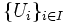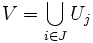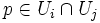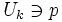# Basis for a topological space

Jump to: navigation, search
This article is about a basic definition in topology.
VIEW: Definitions built on this | Facts about this | Survey articles about this
View a complete list of basic definitions in topology

## Definition when the topological space is specified

### Symbol-free definition

A basis for a topological space is a collection of open subsets of the topological space, such that every open subset can be expressed as a (possibly empty) union of basis subsets.

### Definition with symbols

If$X$ is a topological space, a basis for$X$ is a collection$\{ U_i \}_{i \in I}$ of open subsets of$X$ (here,$I$ is an indexing set) such that for any open subset$V$ of$X$, there exists$J \subset I$ such that:$V = \bigcup_{i \in J} U_j$

## Definition when the topological space is not specified

### Symbol-free definition

Given a set, a collection of subsets of the set is said to form a basis for a topological space or a basis for a topology if the following two conditions are satisfied:

• The union of all members of the collection is the whole space
• Any finite intersection of members of the collection, is itself a union of members of the collection

The topology generated by this basis is the topology in which the open sets are precisely the unions of basis sets.

### Definition with symbols

In symbols: if$X$ is a set, a collection$\{ U_i \}_{i \in I}$ of subsets of$X$ is said to form a basis for a topology on$X$ if the following two conditions are satisfied:

•$\bigcup_{i \in I} U_i = X$
• For all$i, j \in I$, there exists$J \subset I$ such that:$U_i \cap U_j = \bigcap_{k \in J} U_k$

The second condition is sometimes stated as follows: if$p \in U_i \cap U_j$, then there exists$U_k \ni p$ such that$U_k \subset U_i \cap U_j$.

The topology generated by the$U_i$s is defined as follows: a subset$V$ is open in$X$ if and only if there exists

### Equivalence of definitions

Further information: Equivalence of definitions of basis

## Examples

### Extreme examples

1. For any topological space, the collection of all open subsets is a basis. That's because any open subset of a topological space can be expressed as a union of size one.
2. For a discrete topological space, the collection of one-point subsets forms a basis. That's because every open subset of a discrete topological space is a union of one-point subsets, namely, the one-point subsets corresponding to its elements.

### Examples from metric spaces

1. Euclidean space: A basis for the usual topology on Euclidean space is the open balls. An open ball of radius$r > 0$ centered at a point$x$, is defined as the set of all$y \in \R^n$ whose distance from$x$ is strictly smaller than$r$. By the way the topology on$\R^n$ is defined, these open balls clearly form a basis.
2. Metric space: Given any metric space, there is a natural way of viewing it as a topological space. This natural way involves declaring the collection of open balls in the metric space as a basis. To see that this gives a well-defined topology, we need to check that the collection of open balls satisfies the conditions to be a basis for a topological space. This follows from the conditions for a metric space, including nonnegativity, symmetry and the triangle inequality.